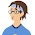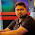## Viral Challenge - Share when you solve it

Glass and water puzzle, how fast can you solve it? Find the value of ?

Solving these type of mathematical puzzles will help you in competitive exams and interviews.
If you couldn't solve, check for the correct answer with explanation below.

Explanation
Let us assume that:

B = half full Blue glass
Y = half full Yellow glass
R = half full Red glass

Therefore,
2B + B + 2B = 15
That is, 5B = 15
So, B = 3

2Y – Y + 2B = 10
That is, Y + 2x3 = 10
So, Y = 4

2R + 2R – R = 6
That is, 3R = 6
So, R = 2

So the last equation becomes,
B + (B+R)xY = 3 + (3+2)x4
That is, 3 + 20 = 23

1.Nice website. keep it up.Anyway thanks again.

regards,
envatocanyon

2.Thank you for the great post really it help me.

3.You discussed this topic very easily as you do always. Keep up this great job.

Thanks
Mhreja
https://www.mastisky.in

4.